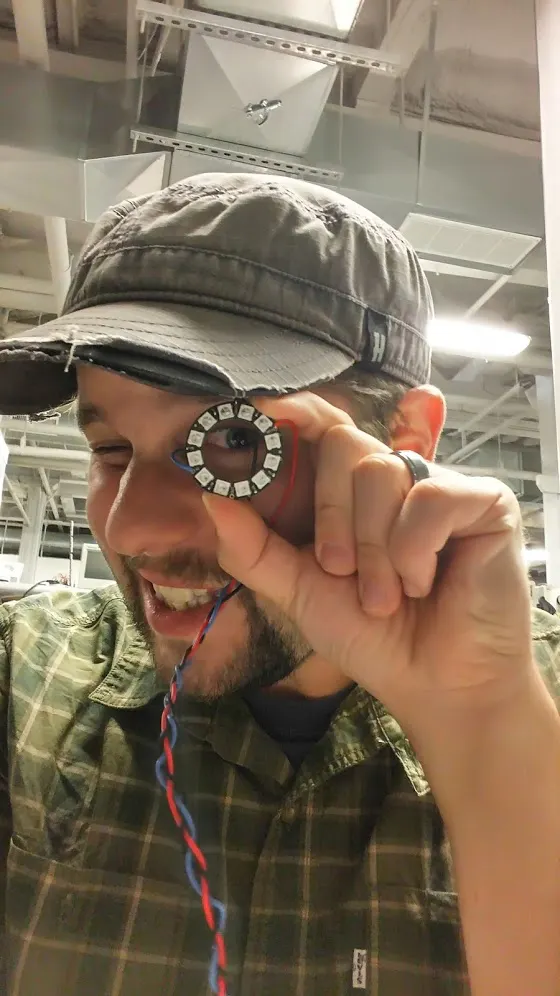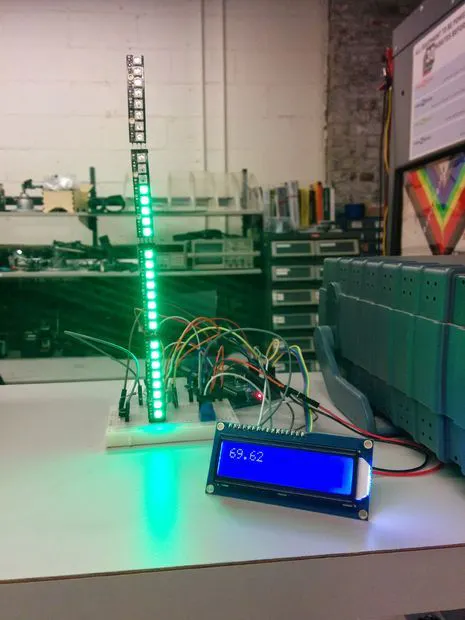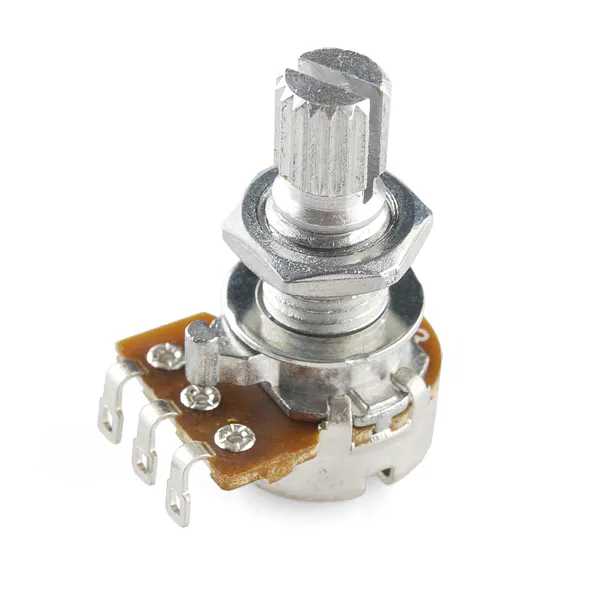Published

# Indoor NeoPixel Thermometer

An indoor thermometer with LCD and "analog" readouts.

IntermediateFull instructions provided11,455## Things used in this project

### Hardware componentsArduino UNO
×1
 NeoPixel
×1
×1
 TMP36 sensor
×1Resistor 1k ohm
×1
 470 ohm resistor
×1Rotary potentiometer (generic)
×1
 Simple momentary switch
×1
 F to M jumper wires for the LCD
×1
 M to M Jumpers for everything else
×1×1

## Code

### file_9790.txt

C/C++
neoTemp.ino
```#include <LiquidCrystal.h>

// Create a variable that will store the temperature value
int TMPin = 0;
const int button = 12;
int lastColor = -1;

#define aref_voltage 3.3
#define PIN 6

/*
Param 1 == number of pixels
Param 2 == Arduino pin attached to din
Param 3 == pixel type flags, add together as needed
NE0_KHZ800 800 KHZ bitstream (avg neopix)
NE0_GRB wired for GRB bitstream
*/
Adafruit_NeoPixel strip = Adafruit_NeoPixel(32, PIN, NEO_GRB + NEO_KHZ800); //call Neopixels, 32 pixels in all, on pin 6, RGB system and 800 KHZ
LiquidCrystal lcd(13, 11, 5, 4, 3, 2); //call LCD display

void setup()
{
lcd.begin(16,2); //initialize LCD screen 16 by 2
strip.begin(); //begin neo pixel strip
strip.show(); //initialize strip
Serial.begin(9600);// serial monitor access
pinMode(button, INPUT); //button for celsius
analogReference(EXTERNAL); //analog reference for use with TMP36
}

void loop()
{
double temperature;
double temp;
double tempF;

temp = tempMeasure(temperature); //call tempMeasure function
tempF = (temp * 9.0/5.0 + 32);  //take reading from tempMeasure and convert to faharenheit

{
Serial.println(temp); //prints in serial monitor
lcd.print(temp); //prints on LCD screen
lcd.setCursor(0,1); //sets cursor
lcd.clear(); //clear LCD after 2 seconds
}

pixelCaseF(tempF); //call pixelCase function that controls lights
Serial.println(tempF); //print in serial
lcd.print(tempF); //print temp in F on LCD
lcd.setCursor(0,1); //set cursor
delay(2000); //take reading every 2 seconds
lcd.clear(); //clear LCD

}//end loop

double tempMeasure(double temperature)
{
// -----------------
// -----------------

double voltage = reading * aref_voltage; //take initial reading and multiply by 3.3 ref voltage for arduino

voltage /= 1024.0; //divide reading by 1024 to map

temperature = (voltage - 0.5) * 100; //converts 10mv per degree with 500 mV offset to (voltage - 500mV) * 100)

return temperature; //return temperature to top level method

}//end tempMeasure

void pixelCaseF(double tempF)
/*
This method controls the neo-pixel for the "analog" readout on the thermometer. With every temperature group, the number of pixels lit changes along with the color
*/

{
int i;
strip.setBrightness(64);
strip.show();

if (tempF >= 90.05)//if above 90 degrees, strip is red and entire strip is lit
{
strip.clear();
for(int i=0;i <= 32;i++)
{
strip.setPixelColor(i, strip.Color(255,0,0));
}
}
else if (tempF < 90.2 && tempF >= 80.05) //if 90 > tempF >= 80 orange and strip is partially lit up to 29th pixel
{
strip.clear();
for(int i=0;i <= 28;i++)
{
strip.setPixelColor(i, strip.Color(255,128,0));
}
}
else if (tempF < 80.02 && tempF >= 70.5)// if 80 > tempF >= 70 yellow-green and strip is lit up to 25th pixel
{
strip.clear();
for(int i = 0; i <= 24; i++)
{
strip.setPixelColor(i,strip.Color(204,255,0));
}
}
else if (tempF < 70 && tempF >= 60.5)// if 70 > tempF >= 60 green and strip is lit up to 21st pixel
{
strip.clear();
for(int i = 0; i<= 20; i++)
{
strip.setPixelColor(i,strip.Color(0,255,0));
}
}
else if (tempF < 60.02 && tempF >= 50.5) //if 60 > tempF >= 50 blue and strip is lit up to 17th pixel
{
strip.clear();
for(int i = 0; i <= 16; i++)
{
strip.setPixelColor(i,strip.Color(0,0,255));
}
}
else if (tempF < 50.02 && tempF >= 40.5) //if 50 > tempF >= 40 aqua and strip is lit to 13th pixel
{
strip.clear();
for(int i = 0; i <= 12; i++)
{
strip.setPixelColor(i, strip.Color(0,255,255));
}
}
else if (tempF < 40.02 && tempF >= 32.5) //if 40 > tempF >= 32 fuschia and strip is lit to 9th pixel
{
strip.clear();
for(int i = 0; i <= 8; i++)
{
strip.setPixelColor(i, strip.Color(153, 51,255));
}
}
else if (tempF < 32.5) //temp < freezing white and strip is lit to 5th pixel
{
strip.clear();
for(i = 0;i <= 4; i++)
{
strip.setPixelColor(i, strip.Color(255,255,255));
}//end for
}

strip.show(); //update color change

}//end pixelCase
```